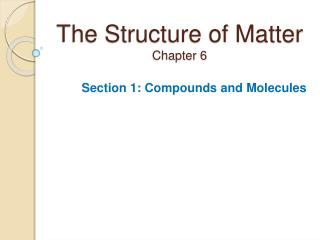DownloadDownload PresentationThe Structure of Matter Chapter 6

# The Structure of Matter Chapter 6

Télécharger la présentation## The Structure of Matter Chapter 6

- - - - - - - - - - - - - - - - - - - - - - - - - - - E N D - - - - - - - - - - - - - - - - - - - - - - - - - - -
##### Presentation Transcript

1. The Structure of MatterChapter 6 Section 1: Compounds and Molecules

2. Bellringer Study the models of the water molecule, H2O, and the carbon dioxide molecule, CO2, and then answer the items that follow. 1. Name some similarities between the molecules of H2O and CO2. 2. How are the molecules different?

3. Chemical Bonds • What holds a compound together? • The forces that hold atoms or ions together in a compound are called chemical bonds. • chemical bond: the attractive force that holds atoms or ions together

4. Atoms and ions are held together by • Nuclear bonds • Stick bonds • Physical bonds • Chemical bonds 30

5. Which of the following is a compound? • Salt • Paint • Soup • Air 30

6. Visual Concept: Compounds

7. Visual Concept: Chemical Bond

8. Chemical Structure • How can the structure of chemical compounds be shown? • The structure of chemical compounds can be shown by various models. Different models show different aspects of compounds. • chemical structure: the arrangement of atoms in a substance

9. Chemical Structure, continued • Some models represent bond lengths and angles. • bond length: the average distance between the nuclei of two bonded atoms • bond angle: the angle formed by two bonds to the same atom

10. Visual Concept: Bond Angle

11. Chemical Structure, continued • In a ball-and-stick model, atoms are represented by balls. The bonds that hold the atoms together are represented by sticks. • In structural formulas, chemical symbols are used to represent the atoms. • Space-filling models show the space occupied by atoms, but not bond lengths.

12. Which of the following models could be used to compare the sizes of atoms in a molecule? • Ball-and-stick • Structural • Space-filling • Formula 30

13. A compound with only two atoms would not have a • Chemical structure • Chemical bond • Bond length • Bond angle 30

14. Which of the following would show bond angle? • Ball-and-stick • Structural • Space-filling • formula 30

15. BELLRINGER #2 • Name and Describe the three kinds of ways we can show chemical structure.

16. Chemical Structure, continued • In a ball-and-stick model, atoms are represented by balls. The bonds that hold the atoms together are represented by sticks. • In structural formulas, chemical symbols are used to represent the atoms. • Space-filling models show the space occupied by atoms, but not bond lengths.

17. Chemical Structure, continued • Bonds can bend, stretch, and rotate without breaking. • Bonds can be represented by flexible springs. • Most reported bond lengths are average distances. • Bonds hold atoms together tightly.

18. How Does Structure Affect Properties? • What determines the properties of a compound? • The chemical structure of a compound determines the properties of that compound.

19. How Does Structure Affect Properties? continued • Compounds with network structures are strong solids. • Example: Quartz, SiO2 is made of silicon and oxygen atoms. • The atoms are bonded in a strong, rigid structure.

20. There are strong bonds between the atoms in a piece of quartz. These bonds give quartz a • Low melting point • High melting point • Low molecular mass • High molecular mass 30

21. How Does Structure Affect Properties?continued • Some networks are made of bonded ions. • The strong attractions between the oppositely charged ions give ionic compounds high melting points and high boiling points. • Example: Table salt—sodium chloride—is made of a tightly packed repeating network of positive sodium ions and negative chlorine ions.

22. How Does Structure Affect Properties? continued • Some compounds are made of molecules. • Some compounds made of molecules are solids, others are liquids, others are gases. • The strength of attractions between molecules varies. • Attractions between water molecules are called hydrogen bonds. • Hydrogen bonds are not as strong as the bonds holding oxygen and hydrogen atoms together within a molecule.

23. The attraction between molecules tends to be ____ the attraction between ions. • Weaker than • Stronger than • Equal to • None of the above 30

24. Which molecules are most strongly attracted to one another? • Water molecules • Hydrogen molecules • Oxygen molecules • Nitrogen molecules 30

25. Sugar has a lower melting point than salt because sugar is made of • Atoms • Ions • Molecules • Crystals 30

26. A hydrogen bond BETWEEN water molecules is which of the following when compared to the bonds within an individual water molecule? • It is stronger • It is weaker • It is the same strength • None of the above 30

27. Participant Scores

28. For Homework: Section Review p. 182 (1-6)